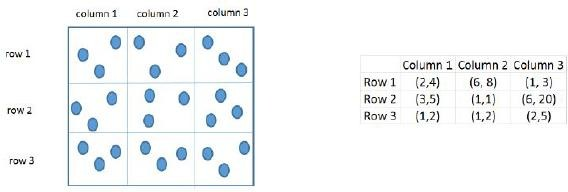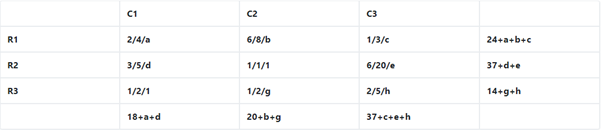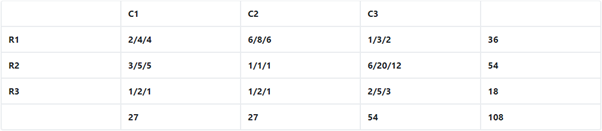# Three pouches (each represented by a filled circle) | CAT DILR questions – Logical Reasoning

## CAT 2019 - Slot 2 - Set 5 - Three pouches (each represented by a filled circle)

#####Three pouches (each represented by a filled circle) are kept in each of the nine slots in a 3× 3 grid, as shown in the figure. Every pouch has a certain number of one-rupee coins. The minimum and maximum amounts of money (in rupees) among the three pouches in each of the nine slots are given in the table. For example, we know that among the three pouches kept in the second column of the first row, the minimum amount in a pouch is Rs. 6 and the maximum amount is Rs. 8. There are nine pouches in any of the three columns, as well as in any of the three rows. It is known that the average amount of money (in rupees) kept in the nine pouches in any column or in any row is an integer. It is also known that the total amount of money kept in the three pouches in the first column of the third row is Rs. 4.

Q. 1: What is the total amount of money (in rupees) in the three pouches kept in the first column of the second row?

Explanation:

If assume the unknown number with a variable , following table can be formed.Minimum value of a+b+c = (2+6+1) = 9 ( when all of a, b and c are minimum possible)

Maximum value of (a+b+c) = (4+8+3) = 15 ( when all of a, b and c are maximum possible)

Similarly Range ( Minimum and Maximum value) of d+e = (9, 25) and g+h = (3, 7)

As sum of all rows and all columns must be multiple of 3 . So on solving we can see a+b+c = 12, d+e = 17 and g+h = 4

Now

Minimum value of a+d = (2+3) = 5 and maximum value of a+d = 4+5 =9

As sum of C1 = 18 + a +d

So a+d should be divisible by 3 . thus a+d can be either 6 or 9.

Similarly b+g can be 7 or 10. And c+h+e can be of the form 3k + 2

On solving we will see only a+d = 9 will satisfy all the conditions

a+d = 9 means a = 4 and d = 5

e = 12. -> c +h = 5. -> b+c =8

if h =3, then c=2, b=6, g=1

Finally, we getNow all the question can be answered.

##### Q. 2: How many pouches contain exactly one coin?

Q. 3: What is the number of slots for which the average amount (in rupees) of its three pouches is an integer?

Q. 4: The number of slots for which the total amount in its three pouches strictly exceeds Rs. 10 is

1.13

2. 8

3. 2

4. 3

Explanation:
If assume the unknown number with a variable , following table can be formed.Minimum value of a+b+c = (2+6+1) = 9 ( when all of a, b and c are minimum possible)

Maximum value of (a+b+c) = (4+8+3) = 15 ( when all of a, b and c are maximum possible)

Similarly Range ( Minimum and Maximum value) of d+e = (9, 25) and g+h = (3, 7)

As sum of all rows and all columns must be multiple of 3 . So on solving we can see a+b+c = 12, d+e = 17 and g+h = 4

Now

Minimum value of a+d = (2+3) = 5 and maximum value of a+d = 4+5 =9

As sum of C1 = 18 + a +d

So a+d should be divisible by 3 . thus a+d can be either 6 or 9.

Similarly b+g can be 7 or 10. And c+h+e can be of the form 3k + 2

On solving we will see only a+d = 9 will satisfy all the conditions

a+d = 9 means a = 4 and d = 5

e = 12. -> c +h = 5. -> b+c =8

if h =3, then c=2, b=6, g=1

Finally, we getNow all the question can be answered.

### Past Year Question Paper & SolutionsCounselling Session
By IIM Mentor

### Free Material Area